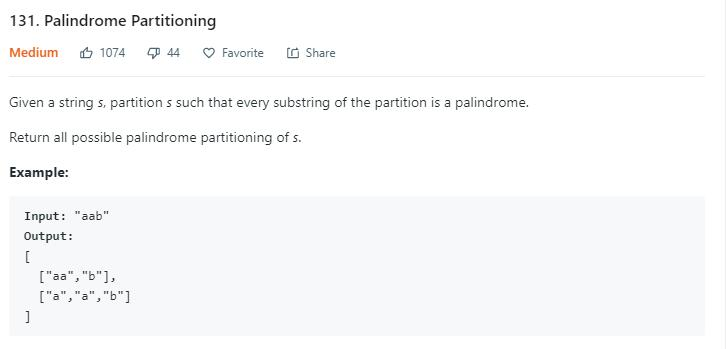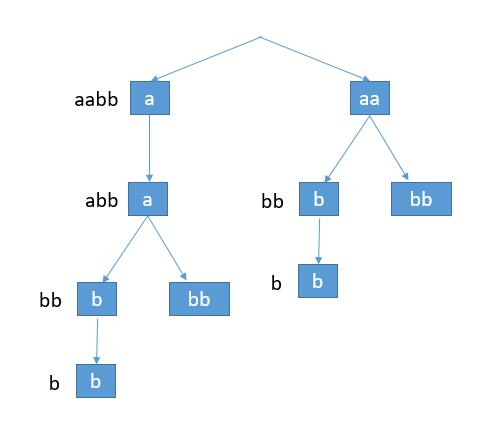# 题目描述（中等难度）# 解法一 分治

aabb

abb 的所有结果就是 [a b b] [a bb]

aabb

bb 的所有结果就是 [b b] [bb]

aabb

aabb

[a a b b] [a a bb] [aa b b] [aa bb]


public List<List<String>> partition(String s) {
return partitionHelper(s, 0);
}

private List<List<String>> partitionHelper(String s, int start) {
//递归出口，空字符串
if (start == s.length()) {
List<String> list = new ArrayList<>();
List<List<String>> ans = new ArrayList<>();
return ans;
}
List<List<String>> ans = new ArrayList<>();
for (int i = start; i < s.length(); i++) {
//当前切割后是回文串才考虑
if (isPalindrome(s.substring(start, i + 1))) {
String left = s.substring(start, i + 1);
//遍历后边字符串的所有结果，将当前的字符串加到头部
for (List<String> l : partitionHelper(s, i + 1)) {
}
}

}
return ans;
}

private boolean isPalindrome(String s) {
int i = 0;
int j = s.length() - 1;
while (i < j) {
if (s.charAt(i) != s.charAt(j)) {
return false;
}
i++;
j--;
}
return true;
}


# 解法二 分治优化

dp[i][j] 表示 s[i，j] 是否是回文串。

boolean[][] dp = new boolean[s.length()][s.length()];
int length = s.length();
//考虑所有长度的子串
for (int len = 1; len <= length; len++) {
//从每个下标开始
for (int i = 0; i <= s.length() - len; i++) {
int j = i + len - 1;
dp[i][j] = s.charAt(i) == s.charAt(j) && (len < 3 || dp[i + 1][j - 1]);
}
}


i + 1 <= j - 1

i + 1 <= i + len - 1 - 1

len >= 3


public List<List<String>> partition(String s) {
boolean[][] dp = new boolean[s.length()][s.length()];
int length = s.length();
for (int len = 1; len <= length; len++) {
for (int i = 0; i <= s.length() - len; i++) {
int j = i + len - 1;
dp[i][j] = s.charAt(i) == s.charAt(j) && (len < 3 || dp[i + 1][j - 1]);
}
}
return partitionHelper(s, 0, dp);
}

private List<List<String>> partitionHelper(String s, int start, boolean[][] dp) {
if (start == s.length()) {
List<String> list = new ArrayList<>();
List<List<String>> ans = new ArrayList<>();
return ans;
}
List<List<String>> ans = new ArrayList<>();
for (int i = start; i < s.length(); i++) {
if (dp[start][i]) {
String left = s.substring(start, i + 1);
for (List<String> l : partitionHelper(s, i + 1, dp)) {
}
}

}
return ans;
}


# 解法三 回溯

115 题 中考虑了分治、回溯、动态规划，这道题同样可以用回溯法。

aabbpublic List<List<String>> partition(String s) {
boolean[][] dp = new boolean[s.length()][s.length()];
int length = s.length();
for (int len = 1; len <= length; len++) {
for (int i = 0; i <= s.length() - len; i++) {
dp[i][i + len - 1] = s.charAt(i) == s.charAt(i + len - 1) && (len < 3 || dp[i + 1][i + len - 2]);
}
}
List<List<String>> ans = new ArrayList<>();
partitionHelper(s, 0, dp, new ArrayList<>(), ans);
return ans;
}

private void partitionHelper(String s, int start, boolean[][] dp, List<String> temp, List<List<String>> res) {
//到了空串就加到最终的结果中
if (start == s.length()) {
}
//在不同位置切割
for (int i = start; i < s.length(); i++) {
//如果是回文串就加到结果中
if (dp[start][i]) {
String left = s.substring(start, i + 1);
partitionHelper(s, i + 1, dp, temp, res);
temp.remove(temp.size() - 1);
}

}
}